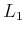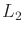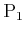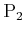#### Nash equilibria in sequential games

Formulations 10.3 and 10.4 can be extended to sequential nonzero-sum games. In the case of game trees, a cost vector, with one element for each player, is written at each of the leaves. Under the stage-by-stage model, deterministic and randomized Nash equilibria can be computed using the bottom-up technique that was presented in Section 10.5.1. This will result in the computation of a single Nash equilibrium. To represent all Nash equilibria is considerably more challenging. As usual, the game tree is decomposed into many matrix games; however, in each case, all Nash equilibria must be found and recorded along with their corresponding costs. Instead of propagating a single cost up the tree, a set of cost vectors, along with the actions associated with each cost vector, must be propagated up the tree to the root. As in the case of a single-stage game, nonadmissible Nash equilibria can be removed from consideration. Thus, from every matrix game encountered in the computation, only the admissible Nash equilibria and their costs should be propagated upward.

Formulation 10.4 can be extended by introducing the cost functionsandforand, respectively. The value-iteration approach can be extended in a way similar to the extension of the game tree method. Multiple value vectors and their corresponding actions must be maintained for each combination of state and stage. These correspond to the admissible Nash equilibria.

The nonuniqueness of Nash equilibria causes the greatest difficulty in the sequential game setting. There are typically many more equilibria in a sequential game than in a single-stage game. Therefore, the concept is not very useful in the design of a planning approach. It may be more useful, for example, in modeling the possible outcomes of a complicated economic system. A thorough treatment of the subject appears in .

Steven M LaValle 2020-08-14